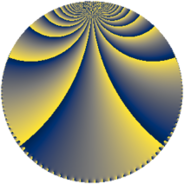# Properties

 Label 1344.4.kLevel $1344$ Weight $4$ Character orbit 1344.k Rep. character $\chi_{1344}(1217,\cdot)$ Character field $\Q$ Dimension $188$ Sturm bound $1024$

# Related objects

## Defining parameters

 Level: $$N$$ $$=$$ $$1344 = 2^{6} \cdot 3 \cdot 7$$ Weight: $$k$$ $$=$$ $$4$$ Character orbit: $$[\chi]$$ $$=$$ 1344.k (of order $$2$$ and degree $$1$$) Character conductor: $$\operatorname{cond}(\chi)$$ $$=$$ $$21$$ Character field: $$\Q$$ Sturm bound: $$1024$$

## Dimensions

The following table gives the dimensions of various subspaces of $$M_{4}(1344, [\chi])$$.

Total New Old
Modular forms 792 196 596
Cusp forms 744 188 556
Eisenstein series 48 8 40

## Trace form

 $$188q - 4q^{9} + O(q^{10})$$ $$188q - 4q^{9} - 52q^{21} + 4292q^{25} + 1016q^{37} - 4q^{49} + 104q^{57} - 2468q^{81} + 1008q^{85} - 104q^{93} + O(q^{100})$$

## Decomposition of $$S_{4}^{\mathrm{new}}(1344, [\chi])$$ into newform subspaces

The newforms in this space have not yet been added to the LMFDB.

## Decomposition of $$S_{4}^{\mathrm{old}}(1344, [\chi])$$ into lower level spaces

$$S_{4}^{\mathrm{old}}(1344, [\chi]) \cong$$ $$S_{4}^{\mathrm{new}}(21, [\chi])$$$$^{\oplus 7}$$$$\oplus$$$$S_{4}^{\mathrm{new}}(42, [\chi])$$$$^{\oplus 6}$$$$\oplus$$$$S_{4}^{\mathrm{new}}(84, [\chi])$$$$^{\oplus 5}$$$$\oplus$$$$S_{4}^{\mathrm{new}}(168, [\chi])$$$$^{\oplus 4}$$$$\oplus$$$$S_{4}^{\mathrm{new}}(336, [\chi])$$$$^{\oplus 3}$$$$\oplus$$$$S_{4}^{\mathrm{new}}(672, [\chi])$$$$^{\oplus 2}$$